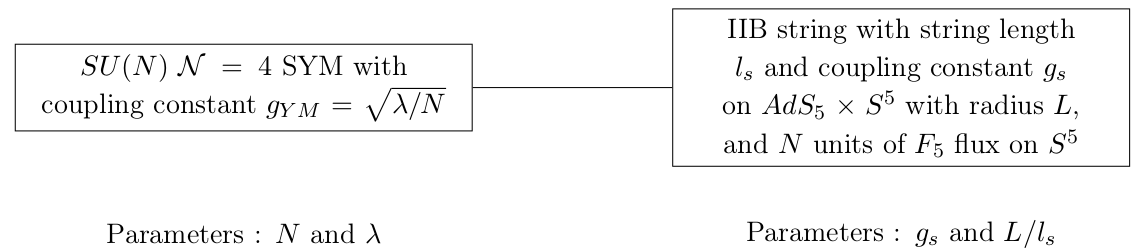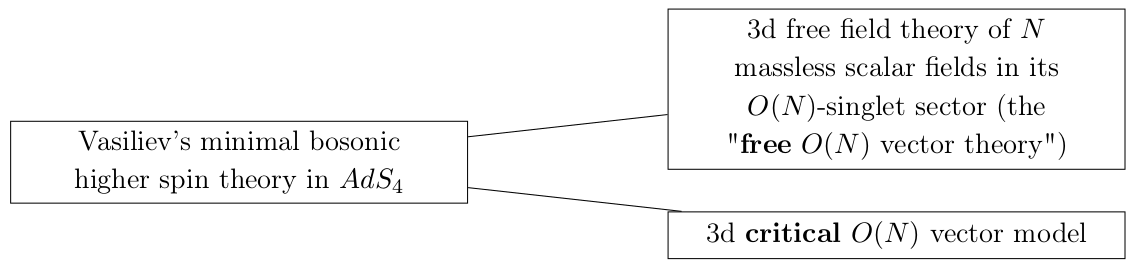Let us first recall the strong form of the $AdS_5/CFT_4$ correspondence in the simplest setup.There are two free parameters on each side, which are related by
\begin{equation}
\frac{\lambda}{N} = 2 \pi g_s \qquad \textrm{and} \qquad 2 \lambda = \left( \frac{L}{l_s}\right)^4 \, .
\end{equation}

Usually one considers the large $N$ and large $\lambda$ limit with small $g_s$ : this relates strongly coupled gauge theory to weakly coupled string theory on large $AdS$ space. In this situation, of course, it is the field theory that is hard to study analytically.

But what happens if we consider instead the large $N$ limit with small $g_s$ but now $\lambda \rightarrow 0$ ? On the gauge theory side, this is a weakly coupled large $N$ theory (which can be understood perturbatively). On the gravity side, this is weakly coupled string theory but on a small $AdS$ space compared to the string length, in other words a strongly coupled sigma model, which is very difficult to study. In this limit, the strings become tensionless (in $AdS$ units), and the string spectrum should contain a tower of higher spin gauge fields. So we are lead to the idea that the gravitational dual of a large $N$ gauge theory in the free limit should by a higher spin theory. In that situation, it is the higher-spin side which is complicated.

In 2002, Klebanov and Polyakov conjectured a relation between theories of infinite number of higher-spin massless gauge fields in $AdS_{d+1}$ and large $N$ $CFT_d$ containing $N$-component vector fields. Let us focus on dimension $d=3$. A substantial evidence for this conjecture was obtained by Giombi and Yin in 2009 by comparing the three-point functions in the two theories. Let us be a bit more precise about the conjecture. The higher-spin theory can be related to two distinct field theories, depending on the choice of boundary condition for the scalar field (which has mass squared $m=2 = -2/L^2$ and dimension of dual operator $\Delta = 1$ for the first line and $\Delta = 2$ for the second):The computation of Giombi and Yin found highly nontrivial agreement of the tree-level 3-point functions of Vasiliev theory with free and critical $O(N)$ vector models at leading order in their $1/N$ expansion. A few months ago, Simone Giombi wrote a nice review of the recent advances in this field of study.